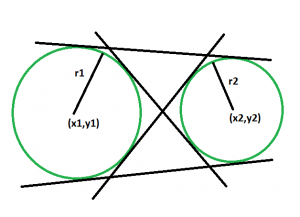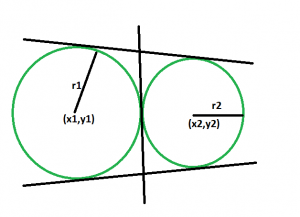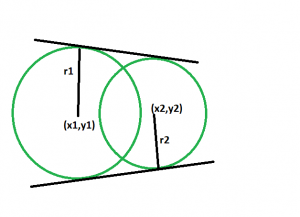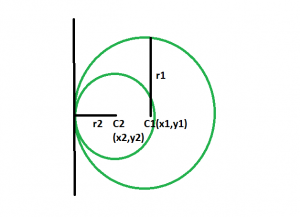# Number of common tangents between two circles if their centers and radius is given

Given two circles with a given radius and centres. The task is to find the number of common tangents between these circles.

Examples:

```Input: x1 = -10, y1 = 8, x2 = 14, y2 = -24, r1 = 30, r2 = 10
Output: 3

Input: x1 = 40, y1 = 8, x2 = 14, y2 = 54, r1 = 39, r2 = 51
Output: 2
```

Approach:

• First of all we will check whether the circles touch each other externally, intersect each other or do not touch each other at all.(Please refer here)
•Then if the circles do not touch each other externally, then obviously they will have 4 common tangents, two direct and two transverse.
•If the circles touch each other externally, then they will have 3 common tangents, two direct and one transverse.
The tangent in between can be thought of as the transverse tangents coinciding together.
•If the circles intersect each other, then they will have 2 common tangents, both of them will be direct.
•If one circle is inside another circle, then they will have only one common tangent.

Below is the implementation of the above approach:

## C++

 `// C++ program to find ` `// the number of common tangents ` `// between the two circles ` ` `  `#include ` `using` `namespace` `std; ` ` `  `int` `circle(``int` `x1, ``int` `y1, ``int` `x2, ` `           ``int` `y2, ``int` `r1, ``int` `r2) ` `{ ` ` `  `    ``int` `distSq = (x1 - x2) * (x1 - x2) ` `                 ``+ (y1 - y2) * (y1 - y2); ` ` `  `    ``int` `radSumSq = (r1 + r2) * (r1 + r2); ` ` `  `    ``if` `(distSq == radSumSq) ` `        ``return` `1; ` `    ``else` `if` `(distSq > radSumSq) ` `        ``return` `-1; ` `    ``else` `        ``return` `0; ` `} ` ` `  `// Driver code ` `int` `main() ` `{ ` `    ``int` `x1 = -10, y1 = 8; ` `    ``int` `x2 = 14, y2 = -24; ` `    ``int` `r1 = 30, r2 = 10; ` `    ``int` `t = circle(x1, y1, x2, ` `                   ``y2, r1, r2); ` `    ``if` `(t == 1) ` `        ``cout << ``"There are 3 common tangents"` `             ``<< ``" between the circles."``; ` `    ``else` `if` `(t < 0) ` `        ``cout << ``"There are 4 common tangents"` `             ``<< ``" between the circles."``; ` `    ``else` `        ``cout << ``"There are 2 common tangents"` `             ``<< ``" between the circles."``; ` ` `  `    ``return` `0; ` `} `

## Java

 `// Java program to find ` `// the number of common tangents ` `// between the two circles ` `import` `java.io.*; ` ` `  `class` `GFG  ` `{ ` `     `  `static` `int` `circle(``int` `x1, ``int` `y1, ``int` `x2, ` `        ``int` `y2, ``int` `r1, ``int` `r2) ` `{ ` ` `  `    ``int` `distSq = (x1 - x2) * (x1 - x2) ` `                ``+ (y1 - y2) * (y1 - y2); ` ` `  `    ``int` `radSumSq = (r1 + r2) * (r1 + r2); ` ` `  `    ``if` `(distSq == radSumSq) ` `        ``return` `1``; ` `    ``else` `if` `(distSq > radSumSq) ` `        ``return` `-``1``; ` `    ``else` `        ``return` `0``; ` `} ` ` `  `// Driver code ` `public` `static` `void` `main (String[] args) ` `{ ` ` `  `    ``int` `x1 = -``10``, y1 = ``8``; ` `    ``int` `x2 = ``14``, y2 = -``24``; ` `    ``int` `r1 = ``30``, r2 = ``10``; ` `    ``int` `t = circle(x1, y1, x2, ` `                ``y2, r1, r2); ` `    ``if` `(t == ``1``) ` `        ``System.out.println (``"There are 3 common tangents"``+ ` `                    ``" between the circles."``); ` `    ``else` `if` `(t < ``0``) ` `        ``System.out.println (``"There are 4 common tangents"``+ ` `            ``" between the circles."``); ` `    ``else` `        ``System.out.println (``"There are 2 common tangents"` `+ ` `                ``" between the circles."``); ` ` `  `     `  `} ` `} ` ` `  `// This code is contributed by ajit.  `

## Python

 `# Python3 program to find ` `# the number of common tangents ` `# between the two circles ` ` `  `def` `circle(x1, y1, x2,y2, r1, r2): ` ` `  ` `  `    ``distSq ``=` `(x1 ``-` `x2) ``*` `(x1 ``-` `x2)``+` `(y1 ``-` `y2) ``*` `(y1 ``-` `y2) ` ` `  `    ``radSumSq ``=` `(r1 ``+` `r2) ``*` `(r1 ``+` `r2) ` ` `  `    ``if` `(distSq ``=``=` `radSumSq): ` `        ``return` `1` `    ``elif` `(distSq > radSumSq): ` `        ``return` `-``1` `    ``else``: ` `        ``return` `0` ` `  ` `  `# Driver code ` `x1,y1 ``=` `-``10``,``8``; ` `x2,y2 ``=` `14``,``-``24``; ` `r1,r2 ``=` `30``,``10``; ` ` `  `t ``=` `circle(x1, y1, x2,y2, r1, r2); ` ` `  `if` `(t ``=``=` `1``): ` `    ``print``(``"There are 3 common tangents between the circles."``) ` `elif` `(t < ``0``): ` `    ``print``(``"There are 4 common tangents between the circles."``) ` `else``: ` `    ``print``(``"There are 2 common tangents between the circles."``) ` ` `  `# This code is contributed by mohit kumar 29 `

## C#

 `// C# program to find  ` `// the number of common tangents  ` `// between the two circles  ` `using` `System; ` ` `  `class` `GFG  ` `{  ` `     `  `static` `int` `circle(``int` `x1, ``int` `y1, ``int` `x2,  ` `        ``int` `y2, ``int` `r1, ``int` `r2)  ` `{  ` ` `  `    ``int` `distSq = (x1 - x2) * (x1 - x2)  ` `                ``+ (y1 - y2) * (y1 - y2);  ` ` `  `    ``int` `radSumSq = (r1 + r2) * (r1 + r2);  ` ` `  `    ``if` `(distSq == radSumSq)  ` `        ``return` `1;  ` `    ``else` `if` `(distSq > radSumSq)  ` `        ``return` `-1;  ` `    ``else` `        ``return` `0;  ` `}  ` ` `  `// Driver code  ` `public` `static` `void` `Main (String []args)  ` `{  ` ` `  `    ``int` `x1 = -10, y1 = 8;  ` `    ``int` `x2 = 14, y2 = -24;  ` `    ``int` `r1 = 30, r2 = 10;  ` `    ``int` `t = circle(x1, y1, x2,  ` `                ``y2, r1, r2);  ` `    ``if` `(t == 1)  ` `        ``Console.WriteLine (``"There are 3 common tangents"``+  ` `                    ``" between the circles."``);  ` `    ``else` `if` `(t < 0)  ` `        ``Console.WriteLine (``"There are 4 common tangents"``+  ` `            ``" between the circles."``);  ` `    ``else` `        ``Console.WriteLine (``"There are 2 common tangents"` `+  ` `                ``" between the circles."``);  ` ` `  `}  ` `}  ` ` `  `// This code is contributed by Arnab Kundu `

## PHP

 ` ``\$radSumSq``)  ` `        ``return` `-1;  ` `    ``else` `        ``return` `0;  ` `}  ` ` `  `    ``// Driver code  ` `    ``\$x1` `= -10; ``\$y1` `= 8;  ` `    ``\$x2` `= 14; ``\$y2` `= -24;  ` `    ``\$r1` `= 30; ``\$r2` `= 10;  ` `    ``\$t` `= circle(``\$x1``, ``\$y1``, ``\$x2``,  ` `                ``\$y2``, ``\$r1``, ``\$r2``);  ` `    ``if` `(``\$t` `== 1)  ` `        ``echo` `"There are 3 common tangents"` `                ``,``" between the circles."``;  ` `    ``else` `if` `(``\$t` `< 0)  ` `        ``echo` `"There are 4 common tangents"` `            ``, ``" between the circles."``;  ` `    ``else` `        ``echo` `"There are 2 common tangents"` `            ``, ``" between the circles."``;  ` `             `  `    ``// This code is contributed by AnkitRai01 ` `?> `

Output:

```There are 3 common tangents between the circles.
```

Attention reader! Don’t stop learning now. Get hold of all the important DSA concepts with the DSA Self Paced Course at a student-friendly price and become industry ready.

My Personal Notes arrow_drop_upProgram Analyst Trainee,Cognizant

If you like GeeksforGeeks and would like to contribute, you can also write an article using contribute.geeksforgeeks.org or mail your article to contribute@geeksforgeeks.org. See your article appearing on the GeeksforGeeks main page and help other Geeks.

Please Improve this article if you find anything incorrect by clicking on the "Improve Article" button below.